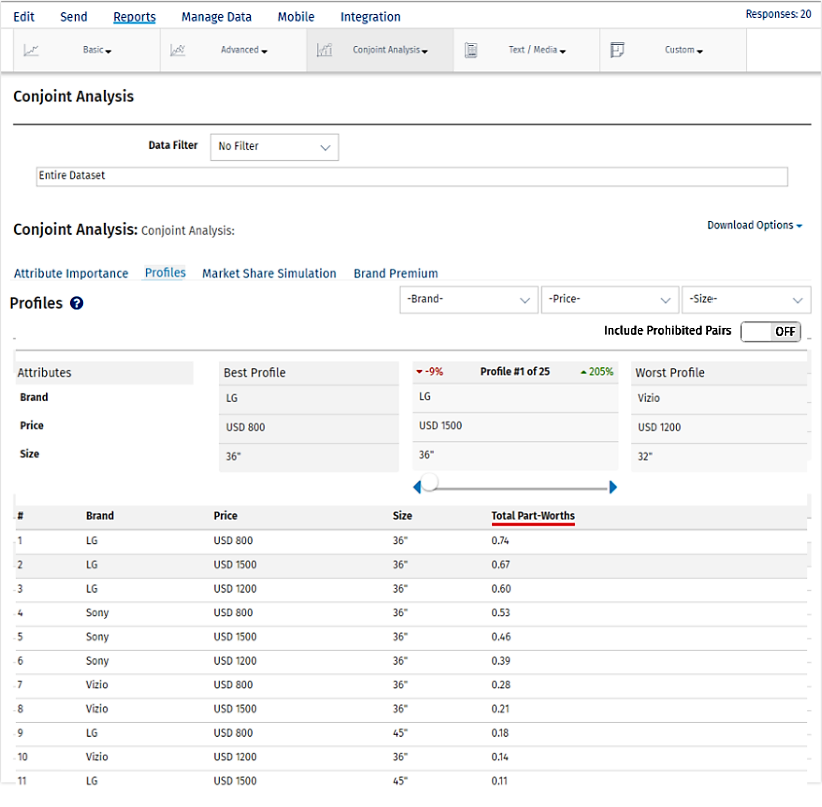# Conjoint Analysis – Profiles

## Profiles

A conjoint profile is a set of attributes with different levels that are displayed for selection. Respondents would be shown a set of products, prototypes, mockups, or pictures created from a combination of levels from all or some of the constituent attributes and asked to choose from the products they are shown. Each example is similar enough that consumers will see them as close substitutes, but dissimilar enough that respondents can clearly determine a preference. Each example is composed of a unique combination of product features.

To view detailed Profiles:

Go to: Login » Surveys » Reports » Choice Modelling » Conjoint Analysis » ProfilesBy default results displayed will be excluding prohibited pairs if any selected by the users in the beginning .

The best profile is always displayed on the left and the worst profile on the right. In between, you can select a custom profile to see how it compares with the best and the worst profile.If any pair is selected in prohibited pair that will not be displayed in best, worst or custom profile.If user still want to see the prohibited pairs they can enable the including prohibited pairs option provided.

For the selected profile, you can see the percentage point difference between the best profile and the selected profile in red.

The percentage point difference between the worst profile and the selected profile is displayed in green.

You can also limit/narrow down on the profiles by selecting specific levels for the attributes.

Attribute and level with highest Total Part Worth Value will be selected as the Best Profile and the one with lowest Total Part Worth value will be selected as the Worst Profile.

Calculating Part-Worths Values

We use the following algorithm to calculate CBC Conjoint Part-Worths:

Notation

Let there be R respondents, with individuals r = 1 ... R

Let each respondent see T tasks, with t = 1 ... T

Let each task t have C configurations (or concepts), with c = 1 ... C (C in our case is usually 3 or 4)

If we have A attributes, a = 1 to A, with each attribute having La levels, l = 1 to La, then the part-worth for a particular attribute/level is w’(a,l). It is this (jagged array) of part worths we are solving for in this exercise.

We can simplify this to a one-dimensional array w(s), where the elements are:{w’(1,1), w’(1,2) ... w’(1,L1), w’(2,1) ... w’(A,LA)} with w having S elements.

A specific configuration x can be represented as a one-dimensional array x(s), where x(s)=1 if the specific level/attribute is present, and 0 otherwise.

Let Xrtc represent the specific configuration of the cth configuration in the tth task for the rth respondent. Thus the experiment design is represented by the four dimensional matrix X with size RxTxCxS

If respondent r chooses configuration c in task t then let Yrtc=1; otherwise 0.

Utility Of A Specific Configuration

The Utility Ux of a specific configuration is the sum of the part-worths for those attribute/levels present in the configuration, i.e. it is the scalar product x.w

The Multinomial Logit Model

For a simple choice between two configurations, with utilities U1 and U2, the MNL model predicts that configuration 1 will be chosen

EXP(U1)/(EXP(U1) + EXP(U2)) of the time (a number between 0 and 1).

For a choice between N configurations, configuration 1 will be chosen

EXP(U1)/(EXP(U1) + EXP(U2) + ... + EXP(UN)) of the time.

Modeled Choice Probability

Let the choice probability (using MNL model) of choosing the cth configuration in the tth task for the rth respondent be:

Prtc=EXP(xrtc.w)/SUM(EXP(xrt1.w), EXP(xrt2.w), ... , EXP(xrtC.w))

Log-likelihood Measure

The Log-Likelihood measure LL is calculated as:Prtc is a function of the part-worth vector w, which is the set of part-worths we are solving for.

Solving For Part-worths Using Maximum Likelihood

We solve for the part-worth vector by finding the vector w that gives the maximum value for LL. Note that we are solving for S variables.

This is a multi-dimensional non-linear continuous maximization problem, and requires a standard solver library. We use the Nelder-Mead Simplex Algorithm.

The Log-Likelihood function should be implemented as a function LL(w, Y, X), and then optimized to find the vector w that gives us a maximum. The responses Y, and the design X are given, and constant for a specific optimization. Initial values for w can be set to the origin 0.

The final part-worths w are re-scaled so that the part-worths for any attribute have a mean of zero, simply by subtracting the mean of the part-worths for all levels of each attribute.

This feature/tools described here are available with the following license(s) :

Enterprise Edition

Unlimited Surveys, Questions